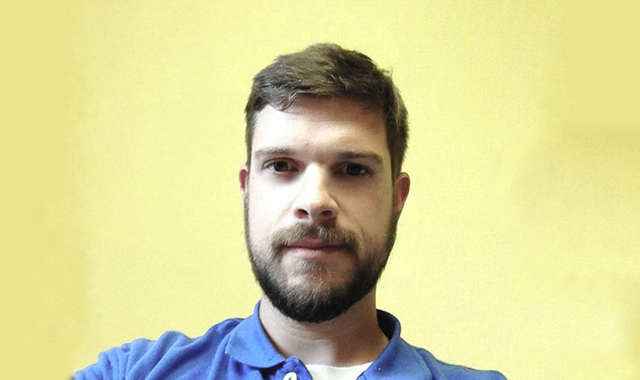# Nature of MathematicsCOURSE NUMBER: MATH 10
INSTRUCTOR: Tim Melvin

## DESCRIPTION

A survey course in mathematical concepts and mathematics in culture. Topics to include mathematical reasoning and four additional topics selected from number theory, probability, statistics, mathematical modeling, contemporary applications, geometry, and the history of mathematics. Recommended for liberal arts students.

## Objectives

Students will be able to:

1. Define inductive reasoning, and apply to patterns and sequences.
2. Define deductive reasoning, and apply to logic and sets.
3. Demonstrate ability to perform four of the following objectives:
• Define various sets of numbers and use number systems of different bases.
• Apply counting techniques, permutations, combinations, and probability models.
• Define frequency distributions and measures of central tendency and dispersion, and create graphical displays of data.
• Apply mathematical models such as linear, quadratic, exponential, and logarithmic, to real-world problems.
• Understand topics within contemporary mathematics, such as voting and apportionment, financial mathematics, graph theory, linear programming, and applications of matrices.
• Define and apply concepts of areas, volumes, Euclidean and non-Euclidean geometry, and selected other topics in geometry.
• Describe the historical development of mathematics, the role of theorem and proof in mathematical thought, and significant mathematical results and mathematicians.